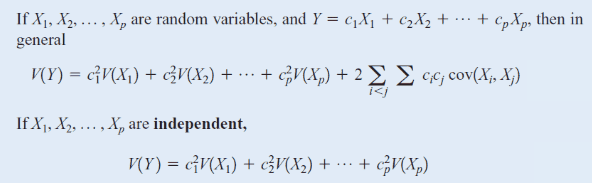# Linear combination of random variables

• archaic
Whoops! I just totally misread the question, sorry. I think my brain just said that doesn't make any sense and converted 10 people into 1000 kilograms.f

#### archaic

Homework Statement
The weight of ##25## individuals trying to squeeze themselves into an elevator is normally distributed with a mean of ##76\,kg## and a standard deviation of ##16\,kg##. The elevator is designed to hold ##1950\,kg##.
a) Determine the mean and standard deviation of the total weight inside the elevator.
b) What's the probability that that weight exceeds the limit?
c) Knowing that the electricity costs ##0.1\,$## per ##kg## for the first ##10## persons, and ##0.2\,$## per ##kg## for any extra person, find the mean and the variance of the cost for this scenario.
Relevant Equations
n/a
a) Total weight ##W=W_1+W_2+...+W_{25}##.$$E[W]=E[W_1]+E[W_2]+...+E[W_{25}]=25\times76=1900\,kg$$$$\sigma_W=\sqrt{V(W_1)+V(W_2)+...+V(W_{25})}=\sqrt{25\times(16)^2}=80\,kg$$
b) Since ##W## is a linear combination of normal distributions, the reproductive property tells us that ##W## is also normally distributed.$$P(W>1950)=P(Z>0.625)=1-P(Z<0.625)=0.26599$$
c) Cost ##C=0.1W_1+...+0.1W_{10}+0.2W_{11}+...+0.2W_{25}##.\begin{align*}E[C]&=0.1E[W_1]+...+0.1E[W_{10}]+0.2E[W_{11}]+...+0.2E[W_{25}]\\&=10\times0.1\times76+15\times0.2\times76\\&=304\,\end{align*}\begin{align*} V(C)&=(0.1)^2V(W_1)+...+(0.1)^2V(W_{10})+(0.2)^2V(W_{11})+...+(0.2)^2V(W_{25})\\ &=10\times(0.1)^2\times(16)^2+15\times(0.2)^2\times(16)^2\\ &=179.2\,^2 \end{align*}
Correct, right? Thanks!

•Delta2
Part (c) is done incorrectly I think. To give an extreme example to demonstrate the issue, suppose electricity cost nothing for the first 1900 kg and cost 20 cents per kilogram after. What would the expected cost of the electricity be?
In practice I think the number you computed for the e.v. is going to be very close to the true answer.

Part (c) is done incorrectly I think. To give an extreme example to demonstrate the issue, suppose electricity cost nothing for the first 1900 kg and cost 20 cents per kilogram after. What would the expected cost of the electricity be?
In practice I think the number you computed for the e.v. is going to be very close to the true answer.
Then we expect the cost to be zero since the expected mass in the elevator is 1900 kg. But isn't this a different scenario? They specified that the cost is per person.
I am sorry, I don't see the point.

Part (c) is done incorrectly I think. To give an extreme example to demonstrate the issue, suppose electricity cost nothing for the first 1900 kg and cost 20 cents per kilogram after. What would the expected cost of the electricity be?
In practice I think the number you computed for the e.v. is going to be very close to the true answer.
Well, it is not stated as so much per kg for the first so many kg; it is for the first so many persons. That's a bit of a problem because we could arrange to have the heaviest get in first!
But I agree that it needs to be approached from first principles: find ##E[C^2]## etc.

Well, it is not stated as so much per kg for the first so many kg; it is for the first so many persons. That's a bit of a problem because we could arrange to have the heaviest get in first!
But I agree that it needs to be approached from first principles: find ##E[C^2]## etc.
What is the significance of the square? Why is it a first principle?

What is the significance of the square? Why is it a first principle?
It's part of the definition of variance: ##E[X^2]-E[X]^2##. Use that to find the variance of the cost.

It's part of the definition of variance: ##E[X^2]-E[X]^2##. Use that to find the variance of the cost.
Oh, ok. I thought that you had some thing in mind that I didn't know like in the other post (minimizing the expression you wrote, and finding ##R^2##).
This is from the lecture slides:I forgot to mention it, but the ##W_i## are identical and independent, so the double sum is null. My bad..

•Delta2
Oh, ok. I thought that you had some thing in mind that I didn't know like in the other post (minimizing the expression you wrote, and finding ##R^2##).
This is from the lecture slides:
View attachment 271835
I forgot to mention it, but the ##W_i## are identical and independent, so the double sum is null. My bad..
Then it's fine.

•archaic
Well, it is not stated as so much per kg for the first so many kg; it is for the first so many persons. That's a bit of a problem because we could arrange to have the heaviest get in first!
But I agree that it needs to be approached from first principles: find ##E[C^2]## etc.

Whoops! I just totally misread the question, sorry. I think my brain just said that doesn't make any sense and converted 10 people into 1000 kilograms.